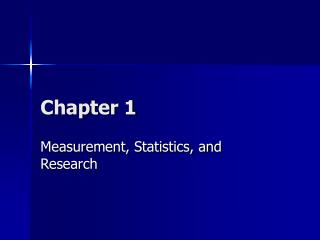DownloadDownload PresentationChapter 1

# Chapter 1

Download Presentation## Chapter 1

- - - - - - - - - - - - - - - - - - - - - - - - - - - E N D - - - - - - - - - - - - - - - - - - - - - - - - - - -
##### Presentation Transcript

1. Chapter 1 Measurement, Statistics, and Research

2. What is Measurement? • Measurement is the process of comparing a value to a standard • Statistics is a mathematical tool used for interpretation • Precision is essential: if the measurement is not PRECISE, the results cannot be TRUSTED

3. What is Measurement? • To be acceptable the data must be • Valid, Reliable & objective • Valid (must be compared to known value or method) • Reliable – is the measurement consistent? • Objective – free from BIAS?

4. Steps in Measurement Process • Object to be measured is identified and defined • The standard to which the object is to be compared is identified and defined • A comparison of the object to the standard is made • A quantitative statement of the relationship between the standard an object is made (statistical evaluation)

5. Variables and Constants • A variable is a characteristic that can assume more than one value • A constant can assume only one value

6. Types of Variables • Continuous variable – can assume any value (ht, wt) • Discrete variable – limited to certain values: integers or whole numbers (2.5 children?)

7. Classification of Data or Level of Measurement • Nominal Scale: mutually exclusive (male, female) • Ordinal Scale: gives quantitative order to the variable, but it DOES NOT indicate how much better one score is than another (RPE of 2 is not twice of 1) • Interval Scale: has equal units and zero is not an absence of the variable (temperature) • Ratio Scale: based on order, has equal distance between scale points, and zero is an absence of value

8. Research Design & Statistical Analysis • Research is a technique for solving problems. Identifying the problem is critical • Types of Research: • Historical • Descriptive • Experimental: involves manipulating and controlling variables to solve a problem • Hypothesis: • an educated guess • based on prior research • Can be tested

9. Hypothesis Testing • Research Hypothesis (H1): predicts relationships or differences between groups • Null Hypothesis (H0): predicts NO relationship or differences between groups • The statistical analysis reports the PROBABILITY that the results would if H0 were true • If the probability (1 in 100) or (5 in 100) that the null is true, we REJECT the null and ACCEPT H1 • NOTE: We never PROVED EITHER!

10. Independent & Dependent Variables • Independent Variable: totally free to vary. (balance is independent of VO2) • Dependent Variable: NOT free to vary (ht and wt) • The INDEPENDENT VARIABLE is controlled by the researcher (effects of exercise on body fat) • The DEPENDENT VARIABLE is the variable being studied (effects of exercise on body fat)

11. Internal Validity • Internal Validity: • refers to the design of the study • All potential intervening variables must be controlled (rat studies are easier to control) • Failure to use a control group harms internal validity • Instrument Error reduces internal validity • Investigator Bias reduces internal validity

12. External Validity • External Validity refers to the ability to generalize the results of a SAMPLE to the POPULATION (rat studies don’t always generalize to humans) • If a sample is not RANDOM it may not represent the population • The process of generalizing from a SAMPLE to a POPULATION is statistical inference

13. Statistical Inference • A Population is a group with a common characteristic • A population is usually large and it is difficult to measure all members • To make inference about a population we take a representative sample (RANDOM) • In a random sample each member of the population is equally likely to be selected • A stratified sample is a sample that is selected according to existing subcategories (rep, dem, ind) • A sample cannot accurately represent the population unless it is drawn without BIAS • In a bias free sample selection of one member does not affect to selection of future subjects

14. Parameters and Statistics • A parameter represents the population • A statistic represents the sample • The difference between a statistic and a parameter is the result of sampling error

15. Probability and Hypothesis Testing • Statistics is the science of making educated guesses • Statistics allow us to make a statement and then cite the odds that it is correct • A random sample of 200 females have a mean ht of 5’ 2” ± 2”. The odds are 95 to 5 that this mean is correct.

16. Probability and Hypothesis Testing • A random sample of 200 females have a mean ht of 5’ 2” ± 2”. • This means that the odds are 95 to 5 that the true mean is between 5’ and 5’ 4” • If a sample results in a mean of 5’ 3” we accept a hypothesis that the ht is 5’ 3” because it lies within the limits (5’ and 5’ 4”)

17. Theories and Hypotheses • A theory is a belief regarding a concept or a series of related concepts • Many hypotheses can be TESTED • If a sufficient number of results confirm the theory it is accepted as true • Mental practice improves performance

18. Misuse of Statistics • Abdominal Exercise devices? • Toothpaste? • Examples of statistics that may or may not be true • Lack of random sample, small sample size, research is PAID • Outliers: extreme scores (more than 3 SD) • Mean income (Income is a skewed distribution)